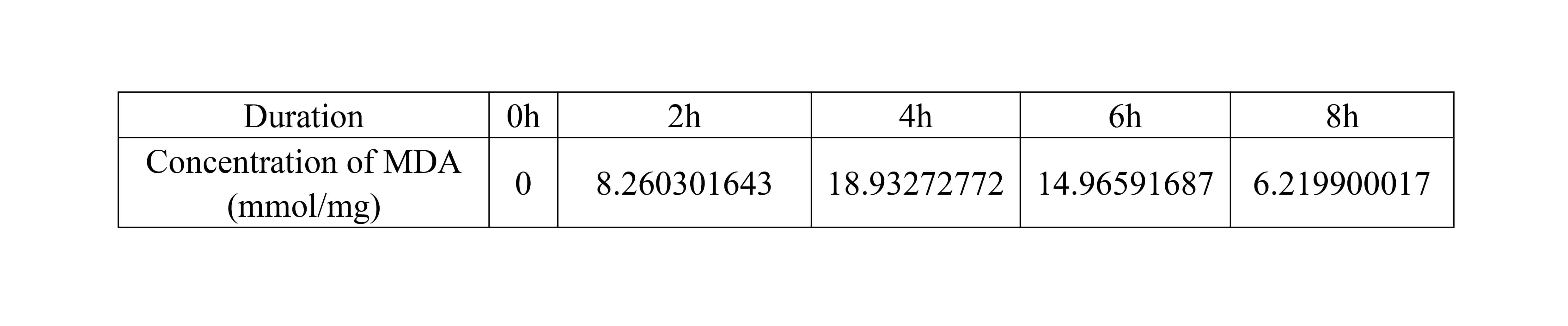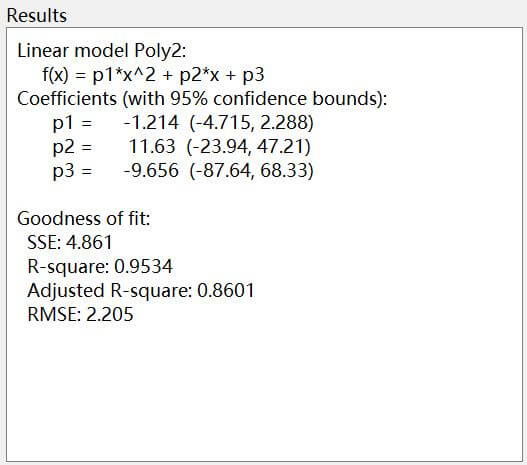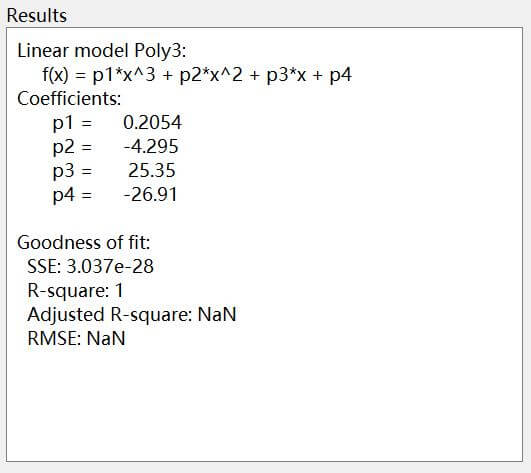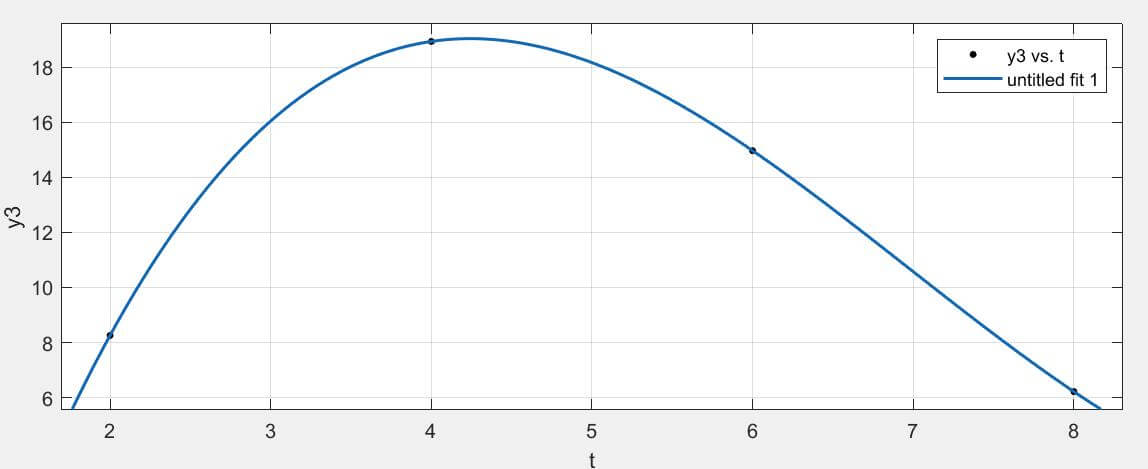# Team:The Webb Schools/Model

The_Webb_Schools

Model
In order to further monitor the performance of our engineered strains, we measured the concentration of MDA in bacteria C (efeB-amilGFP) system during 8 hours where the initial concentration of H2O2 was 2.5mM.
Table 1. the concentration of MDA in bacteria C systemFor quantifying the relationship between the concentration of MDA and the hours in bacteria C system, we firstly attempted to use the quadratic function y = at2 + bt + c which is usually applied in the kinetic models of chemical reactions to fit our data. However, the fitting degree of the quadratic function is only 0.95 which is quite lower than our expectation as showing in the figure 1.Figure 1. Modeling results of y = at2 + bt + c
Therefor, we decided to adopt our data to the cubic function:
y = at3 + bt2 + ct + d
Following are the modeling results with a surprising fitting degree, 1 which could indicate the relationship between the MDA level and the time duration is more likely tending to the cubic function model.Figure 2. Modeling results of y = at3 + bt2 + ct + dFigure 3. The curve of y = at3 + bt2 + ct + d model
According to the function that we solved to fit the relationship model between the concentration of MDA and the hours in bacteria C system, we could see that the MDA level starts to decrease in 4 hours around after the strain enters into the system. In that moment, the inhibition capacity of our engineered strain which could secret efeB enzyme exceeded the generative capacity of MDA. Once we collect more data regarding various concentration of H2O2, we could adjust them to this model to further determine the hours as a reference that our product would take effects in human body with different levels of depression (oxidation).